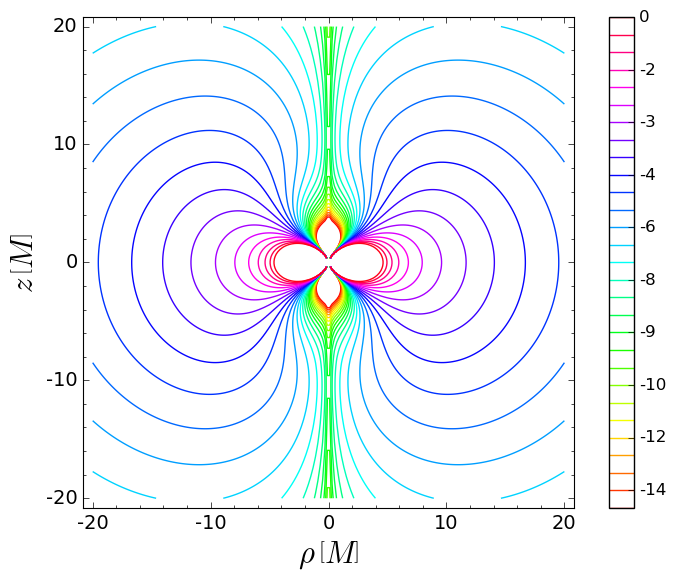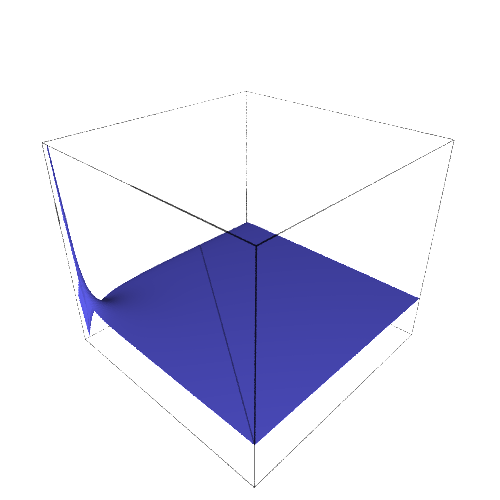# Simon-Mars tensor in Curzon-Chazy spacetime¶

This notebook demonstrates a few capabilities of SageMath in computations regarding Curzon-Chazy spacetime. It implements the computation of the Simon-Mars tensor of Curzon-Chazy spacetime used in the article arXiv:1412.6542. The corresponding tools have been developed within the SageManifolds project (version 1.3, as included in SageMath 8.3).

Click here to download the notebook file (ipynb format). To run it, you must start SageMath with the Jupyter notebook, via the command sage -n jupyter

NB: a version of SageMath at least equal to 8.2 is required to run this notebook:

In :
version()

Out:
'SageMath version 8.3, Release Date: 2018-08-03'

First we set up the notebook to display mathematical objects using LaTeX rendering:

In :
%display latex


## Spacetime manifold¶

We declare the Curzon-Chazy spacetime as a 4-dimensional Lorentzian manifold:

In :
M = Manifold(4, 'M', latex_name=r'\mathcal{M}', structure='Lorentzian')
print(M)

4-dimensional Lorentzian manifold M


We introduce the coordinates $(t,r,y,\phi)$ with $y$ related to the standard Weyl-Papapetrou coordinates $(t,r,\theta,\phi)$ by $y=\cos\theta$:

In :
X.<t,r,y,ph> = M.chart(r't r:(0,+oo) y:(-1,1) ph:(0,2*pi):\phi')
print(X) ; X

Chart (M, (t, r, y, ph))

Out:

## Metric tensor

We declare the only parameter of the Curzon-Chazy spacetime, which is the mass $m$ as a symbolic variable:

In :
var('m')

Out:

Without any loss of generality, we set $m$ to some specific value (this amounts simply to fixing some length scale):

In :
m = 12


Let us set the components of the spacetime metric in the coordinate frame associated with Weyl-Papapetrou coordinates:

In :
g = M.metric()
g[0,0] = - exp(-2*m/r)
g[1,1] = exp(2*m/r-m^2*(1-y^2)/r^2)
g[2,2] = exp(2*m/r-m^2*(1-y^2)/r^2)*r^2/(1-y^2)
g[3,3] = exp(2*m/r)*r^2*(1-y^2)

In :
g[:]

Out:

The Levi-Civita connection $\nabla$ associated with $g$:

In :
nab = g.connection() ; print(nab)

Levi-Civita connection nabla_g associated with the Lorentzian metric g on the 4-dimensional Lorentzian manifold M


As a check, we verify that the covariant derivative of $g$ with respect to $\nabla$ vanishes identically:

In :
nab(g).display()

Out:

## Killing vector

The default vector frame on the spacetime manifold is the coordinate basis associated with Weyl-Papapetrou coordinates:

In :
M.default_frame() is X.frame()

Out:
In :
X.frame()

Out:

Let us consider the first vector field of this frame:

In :
xi = X.frame() ; xi

Out:
In :
print(xi)

Vector field d/dt on the 4-dimensional Lorentzian manifold M


The 1-form associated to it by metric duality is

In :
xi_form = xi.down(g)
xi_form.set_name('xi_form', r'\underline{\xi}')
print(xi_form) ; xi_form.display()

1-form xi_form on the 4-dimensional Lorentzian manifold M

Out:

Its covariant derivative is

In :
nab_xi = nab(xi_form)
print(nab_xi) ; nab_xi.display()

Tensor field nabla_g(xi_form) of type (0,2) on the 4-dimensional Lorentzian manifold M

Out:

Let us check that the Killing equation is satisfied:

In :
nab_xi.symmetrize().display()

Out:

Equivalently, we check that the Lie derivative of the metric along $\xi$ vanishes:

In :
g.lie_der(xi).display()

Out:

Thank to Killing equation, $\nabla_g \underline{\xi}$ is antisymmetric. We may therefore define a 2-form by $F := - \nabla_g \xi$. Here we enforce the antisymmetry by calling the function antisymmetrize() on nab_xi:

In :
F = - nab_xi.antisymmetrize()
F.set_name('F')
print(F)
F.display()

2-form F on the 4-dimensional Lorentzian manifold M

Out:

We check that

In :
F == - nab_xi

Out:

The squared norm of the Killing vector is

In :
lamb = - g(xi,xi)
lamb.set_name('lambda', r'\lambda')
print(lamb)
lamb.display()

Scalar field lambda on the 4-dimensional Lorentzian manifold M

Out:

Instead of invoking $g(\xi,\xi)$, we could have evaluated $\lambda$ by means of the 1-form $\underline{\xi}$ acting on the vector field $\xi$:

In :
lamb == - xi_form(xi)

Out:

or we could have used index notation in the form $\lambda = - \xi_a \xi^a$:

In :
lamb == - ( xi_form['_a']*xi['^a'] )

Out:

## Curvature

The Riemann curvature tensor associated with $g$ is

In :
Riem = g.riemann()
print(Riem)

Tensor field Riem(g) of type (1,3) on the 4-dimensional Lorentzian manifold M


The component $R^0_{\ \, 101} = R^t_{\ \, rtr}$ is

In :
Riem[0,1,0,1]

Out:

while the component $R^2_{\ \, 323} = R^y_{\ \, \phi y \phi}$ is

In :
Riem[2,3,2,3]

Out:

All the non-vanishing components of the Riemann tensor, taking into account the antisymmetry on the last two indices:

In :
Riem.display_comp(only_nonredundant=True)

Out:

The Ricci tensor:

In :
Ric = g.ricci()
print(Ric)

Field of symmetric bilinear forms Ric(g) on the 4-dimensional Lorentzian manifold M


Let us check that the Curzon-Chazy metric is a solution of the vacuum Einstein equation:

In :
Ric.display()

Out:

The Weyl conformal curvature tensor is

In :
C = g.weyl()
print(C)

Tensor field C(g) of type (1,3) on the 4-dimensional Lorentzian manifold M


Let us exhibit two of its components $C^0_{\ \, 123}$ and $C^0_{\ \, 101}$:

In :
C[0,1,2,3]

Out:
In :
C[0,1,0,1]

Out:

To form the Mars-Simon tensor, we need the fully covariant (type-(0,4) tensor) form of the Weyl tensor (i.e. $C_{\alpha\beta\mu\nu} = g_{\alpha\sigma} C^\sigma_{\ \, \beta\mu\nu}$); we get it by lowering the first index with the metric:

In :
Cd = C.down(g)
print(Cd)

Tensor field of type (0,4) on the 4-dimensional Lorentzian manifold M


The (monoterm) symmetries of this tensor are those inherited from the Weyl tensor, i.e. the antisymmetry on the last two indices (position 2 and 3, the first index being at position 0):

In :
Cd.symmetries()

no symmetry; antisymmetry: (2, 3)


Actually, Cd is also antisymmetric with respect to the first two indices (positions 0 and 1), as we can check:

In :
Cd == Cd.antisymmetrize(0,1)

Out:

To take this symmetry into account explicitely, we set

In :
Cd = Cd.antisymmetrize(0,1)


Hence we have now

In :
Cd.symmetries()

no symmetry; antisymmetries: [(0, 1), (2, 3)]


## Simon-Mars tensor

The Simon-Mars tensor with respect to the Killing vector $\xi$ is a rank-3 tensor introduced by Marc Mars in 1999 (Class. Quantum Grav. 16, 2507). It has the remarkable property to vanish identically if, and only if, the spacetime $(\mathcal{M},g)$ is locally isometric to a Kerr spacetime.

Let us evaluate the Simon-Mars tensor by following the formulas given in Mars' article. The starting point is the self-dual complex 2-form associated with the Killing 2-form $F$, i.e. the object $\mathcal{F} := F + i \, {}^* F$, where ${}^*F$ is the Hodge dual of $F$:

In :
FF = F + I * F.hodge_dual(g)
FF.set_name('FF', r'\mathcal{F}')
print(FF) ; FF.display()

2-form FF on the 4-dimensional Lorentzian manifold M

Out:

Let us check that $\mathcal{F}$ is self-dual, i.e. that it obeys ${}^* \mathcal{F} = -i \mathcal{F}$:

In :
FF.hodge_dual(g) == - I * FF

Out:

Let us form the right self-dual of the Weyl tensor as follows

$$\mathcal{C}_{\alpha\beta\mu\nu} = C_{\alpha\beta\mu\nu} + \frac{i}{2} \epsilon^{\rho\sigma}_{\ \ \ \mu\nu} \, C_{\alpha\beta\rho\sigma}$$

where $\epsilon^{\rho\sigma}_{\ \ \ \mu\nu}$ is associated to the Levi-Civita tensor $\epsilon_{\rho\sigma\mu\nu}$ and is obtained by

In :
eps = g.volume_form(2)  # 2 = the first 2 indices are contravariant
print(eps)
eps.symmetries()

Tensor field of type (2,2) on the 4-dimensional Lorentzian manifold M
no symmetry; antisymmetries: [(0, 1), (2, 3)]


The right self-dual Weyl tensor is then:

In :
CC = Cd + I/2*( eps['^rs_..']*Cd['_..rs'] )
CC.set_name('CC', r'\mathcal{C}') ; print(CC)

Tensor field CC of type (0,4) on the 4-dimensional Lorentzian manifold M

In :
CC.symmetries()

no symmetry; antisymmetries: [(0, 1), (2, 3)]

In :
CC[0,1,2,3]

Out:

The Ernst 1-form $\sigma_\alpha = 2 \mathcal{F}_{\mu\alpha} \, \xi^\mu$ (0 = contraction on the first index of $\mathcal{F}$):

In :
sigma = 2*FF.contract(0, xi)


Instead of invoking the function contract(), we could have used the index notation to denote the contraction:

In :
sigma == 2*( FF['_ma']*xi['^m'] )

Out:
In :
sigma.set_name('sigma', r'\sigma')
print(sigma) ; sigma.display()

1-form sigma on the 4-dimensional Lorentzian manifold M

Out:

The symmetric bilinear form $\gamma = \lambda \, g + \underline{\xi}\otimes\underline{\xi}$:

In :
gamma = lamb*g + xi_form * xi_form
gamma.set_name('gamma', r'\gamma')
print(gamma) ; gamma.display()

Field of symmetric bilinear forms gamma on the 4-dimensional Lorentzian manifold M

Out:

### Final computation leading to the Simon-Mars tensor:

The first part of the Simon-Mars tensor is

$$S^{(1)}_{\alpha\beta\gamma} = 4 \mathcal{C}_{\mu\alpha\nu\beta} \, \xi^\mu \, \xi^\nu \, \sigma_\gamma$$

In :
S1 = 4*( CC.contract(0,xi).contract(1,xi) ) * sigma
print(S1)

Tensor field of type (0,3) on the 4-dimensional Lorentzian manifold M


The second part is the tensor

$$S^{(2)}_{\alpha\beta\gamma} = - \gamma_{\alpha\beta} \, \mathcal{C}_{\rho\gamma\mu\nu} \, \xi^\rho \, \mathcal{F}^{\mu\nu}$$

which we compute by using the index notation to denote the contractions:

In :
FFuu = FF.up(g)
xiCC = CC['_.r..']*xi['^r']
S2 = gamma * ( xiCC['_.mn']*FFuu['^mn'] )
print(S2)

Tensor field of type (0,3) on the 4-dimensional Lorentzian manifold M

In :
S2.symmetries()

symmetry: (0, 1); no antisymmetry


The Mars-Simon tensor with respect to $\xi$ is obtained by antisymmetrizing $S^{(1)}$ and $S^{(2)}$ on their last two indices and adding them:

$$S_{\alpha\beta\gamma} = S^{(1)}_{\alpha[\beta\gamma]} + S^{(2)}_{\alpha[\beta\gamma]}$$

We use the index notation for the antisymmetrization:

In :
S1A = S1['_a[bc]']
S2A = S2['_a[bc]']


An equivalent writing would have been (the last two indices being in position 1 and 2):

In :
# S1A = S1.antisymmetrize(1,2)
# S2A = S2.antisymmetrize(1,2)


The Simon-Mars tensor is

In :
S = S1A + S2A
S.set_name('S') ; print(S)
S.symmetries()

Tensor field S of type (0,3) on the 4-dimensional Lorentzian manifold M
no symmetry; antisymmetry: (1, 2)

In :
S.display()

Out:
In :
S.display_comp()

Out:

Hence the Simon-Mars tensor is not zero: the Curzon-Chazy spacetime is not locally isomorphic to the Kerr spacetime.

### Computation of the Simon-Mars scalars

First we form the "square" of the Simon-Mars tensor:

In :
Su = S.up(g)
print(Su)

Tensor field of type (3,0) on the 4-dimensional Lorentzian manifold M

In :
SS = S['_ijk']*Su['^ijk']
print(SS)

Scalar field on the 4-dimensional Lorentzian manifold M

In :
SS.display()

Out:
In :
SSE=SS.expr()


Then we take the real and imaginary part of this compex scalar field. Because this spacetime is spherically symmetric, we expect that the imaginary part vanishes.

In :
SS1 = real(SSE) ; SS1

Out:
In :
SS2 = imag(SSE) ; SS2

Out:

Furthermore we scale those scalars by the ADM mass of the Curzon-Chazy spacetime, which corresponds to $m$:

In :
SS1ad = m^6*SS1 ; SS1ad

Out:

And we take the log of this quantity

In :
lSS1ad = log(SS1ad,10) ; lSS1ad

Out:

Then we plot the value of this quantity as a function of $\rho = x = r \sqrt{1-y^2}$ and $z = r y$, thereby producing Figure 10 of arXiv:1412.6542:

In :
var('x z')
y = z/sqrt(x^2+z^2)).simplify_full()

Out:
In :
S1CC1 = contour_plot(lSS1xzad, (x,-20,20), (z,-20,20), plot_points=200,
fill=False, cmap='hsv', linewidths=1,
contours=(-14,-13.5,-13,-12.5,-12,-11.5,-11,
-10.5,-10,-9.5,-9,-8.5,-8,-7.5,-7,
-6.5,-6,-5.5,-5,-4.5,-4,-3.5,-3,-2.5,
-2,-1.5,-1,-0.5,0),
colorbar=True, colorbar_spacing='uniform',
colorbar_format='%1.f',
axes_labels=(r"$\rho\,\left[M\right]$",
r"$z\,\left[M\right]$"),
fontsize=14)
S1CC1

Out:We also a viewer for 3D plots (use 'threejs' or 'jmol' for interactive 3D graphics):

In :
viewer3D = 'tachyon' # must be 'threejs', 'jmol', 'tachyon' or None (default)

In :
plot3d(lSS1xzad, (x,0.12,20), (z,0.12,20), viewer=viewer3D,
aspect_ratio=[1,1,0.05], plot_points=100,
axes_labels=['rho', 'z', 'log(beta)'])

Out: